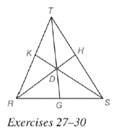Chapter 5.6, Problem 27EElementary Geometry For College St...

7th Edition
Alexander + 2 others
ISBN: 9781337614085

Solutions

Chapter
SectionElementary Geometry For College St...

7th Edition
Alexander + 2 others
ISBN: 9781337614085
Textbook Problem

Given point D in the interior of Δ R S T , which statement(s) is (are) true?a) R K K T ⋅ T H H S ⋅ G S R G = 1 b) T K K R ⋅ R G G S ⋅ S H H T = 1

To determine

a)

To verify:

Whether the statement RKKTTHHSGSRG=1 is true or not.

Explanation

Given:

Consider a point D in the interior of ΔRST.

Theorem used:

Ceva’s theorem:

Let point D be any point in the interior of ΔABC, where E, F, and G lie on ΔABC, let BE¯,AF¯ and CG¯ be the line segments determined by D and vertices of ΔABC

To determine

b)

To verify:

Whether the statement TKKRRGGSSHHT=1 is true or not.

Still sussing out bartleby?

Check out a sample textbook solution.

See a sample solution

The Solution to Your Study Problems

Bartleby provides explanations to thousands of textbook problems written by our experts, many with advanced degrees!

Get Started

Simplify the expressions in Exercises 97106. x3/2x5/2

Finite Mathematics and Applied Calculus (MindTap Course List)

Find f'(x) and f"(x). f(x)=xx21

Single Variable Calculus: Early Transcendentals, Volume I

Evaluate i=1n32i1.

Single Variable Calculus: Early Transcendentals

For

Study Guide for Stewart's Multivariable Calculus, 8th

Given the Taylor Series , a Taylor series for ex/2 is:

Study Guide for Stewart's Single Variable Calculus: Early Transcendentals, 8th